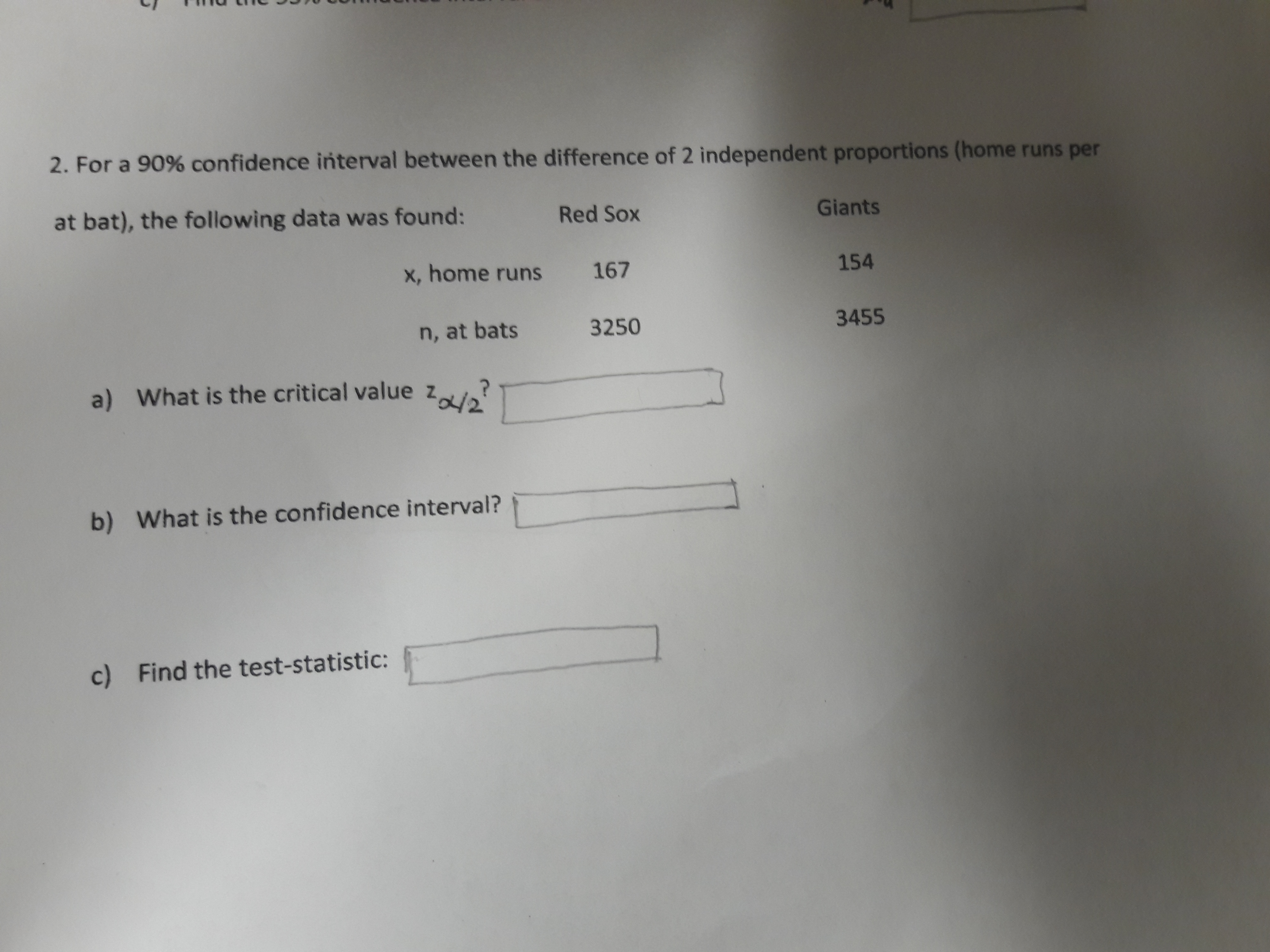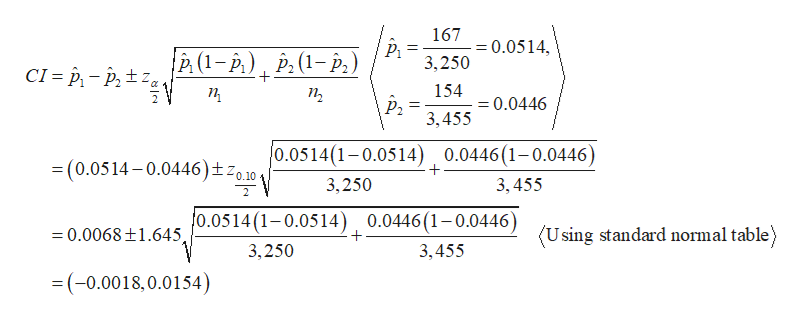# 2. For a 90% confidence interval between the difference of 2 independent proportions (home runs perat bat), the following data was found:Red SoxGiantsx, home runs167154n, at bats32503455a) What is the critical value 2/2b)What is the confidence interval?c)Find the test-statistic:

Question
2 viewshelp_outlineImage Transcriptionclose2. For a 90% confidence interval between the difference of 2 independent proportions (home runs per at bat), the following data was found: Red Sox Giants x, home runs 167 154 n, at bats 3250 3455 a) What is the critical value 2/2 b) What is the confidence interval? c) Find the test-statistic: fullscreen
check_circle

Step 1

It is given that the level of confidence is 0.90.

Step 2

a)

The critical value for the level of confidence 0.90 using standard normal table is 1.645.

b)

...help_outlineImage Transcriptionclose167 = 0.0514, %3D è (1- p) , P.(1-p,) 3,250 CI = p, – P, ±z, 154 п п, = 0.0446 P2 3,455 0.0514(1-0.0514) 0.0446(1-0.0446) = (0.0514 - 0.0446)±zo.10+ 3,250 3, 455 0.0514(1-0.0514) , 0.0446(1-0.0446) = 0.0068±1.645 (Using standard normal table) 3,250 3,455 =(-0.0018,0.0154) fullscreen

### Want to see the full answer?

See Solution

#### Want to see this answer and more?

Solutions are written by subject experts who are available 24/7. Questions are typically answered within 1 hour.*

See Solution
*Response times may vary by subject and question.
Tagged in
MathStatistics